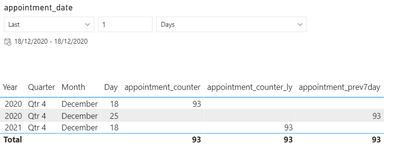cancel
Showing results for
Did you mean:Anonymous
Not applicable

## Different result for date calculation using date slicer with or without hierarchy

Hi,
I've tried searching, but not seen this anywhere...
I'm building a report, and want to keep the date slicer small and simple, so I used a relative date slicer, which also updates to the current date, so that was nice. But when I then add a last year and last week measure, the result is that it will go back in time and show me the current day result. I found this to be linked to the slicer using date hierarchy or not.

Without hierarchy:It seems the calculation goes back in time as requested, then shows the number for the selected date.

With a hierarchy in the slicer:This is the result I was expecting, no matter if the hierarchy was added to the slicer.

Is there anyway to use the relative time slicer and still get the result as with the hierarchy?

Thanks

Øystein

1 ACCEPTED SOLUTIONCommunity Support

Hi @Anonymous ,

If your date column is not continuously, not recommend to use Time-intelligence function like dateadd(), sameperiodlastyear() etc.

Based on your description, you can create a control measure like this, put it in the visual filter and set its value as 1:

``````Control =
VAR _max =
CALCULATE ( MAX ( 'Table'[Date] ), ALLSELECTED ( 'Table' ) )
VAR _min =
CALCULATE ( MIN ( 'Table'[Date] ), ALLSELECTED ( 'Table' ) )
RETURN
IF (
SELECTEDVALUE ( 'Table'[Date] ) >= _min
&& SELECTEDVALUE ( 'Table'[Date] ) <= _max,
1,
0
)``````Then you can create measures like this:

``now = SUM('Table'[value])``
``````prev =
CALCULATE (
SUM ( 'Table'[value] ),
FILTER (
ALL ( 'Table' ),
'Table'[Date]
>= MIN ( 'Table'[Date] ) - 7
&& 'Table'[Date] <= MIN ( 'Table'[Date] )
)
)``````
``````ly =
CALCULATE (
SUM ( 'Table'[value] ),
FILTER (
ALL ( 'Table' ),
YEAR ( 'Table'[Date] )
= YEAR ( MIN ( 'Table'[Date] ) ) - 1
&& QUARTER ( 'Table'[Date] ) = QUARTER ( MIN ( 'Table'[Date] ) )
&& MONTH ( 'Table'[Date] ) = MONTH ( MIN ( 'Table'[Date] ) )
&& DAY ( 'Table'[Date] ) = DAY ( MIN ( 'Table'[Date] ) )
)
)``````Best Regards,
Community Support Team _ Yingjie Li
If this post helps, then please consider Accept it as the solution to help the other members find it more quickly.

4 REPLIES 4Anonymous
Not applicable

Hi,

ØysteinAnonymous
Not applicable

Hi,
sorry, did not add the calculations:

appointment_counter_ly = CALCULATE(sum(sight_tests_details[appointment_counter]),SAMEPERIODLASTYEAR(sight_tests_details[appointment_date].[Date]))

I don't think they're the problem, it's that they behave differently depending on the slicer...

ØysteinCommunity Support

Hi @Anonymous ,

If your date column is not continuously, not recommend to use Time-intelligence function like dateadd(), sameperiodlastyear() etc.

Based on your description, you can create a control measure like this, put it in the visual filter and set its value as 1:

``````Control =
VAR _max =
CALCULATE ( MAX ( 'Table'[Date] ), ALLSELECTED ( 'Table' ) )
VAR _min =
CALCULATE ( MIN ( 'Table'[Date] ), ALLSELECTED ( 'Table' ) )
RETURN
IF (
SELECTEDVALUE ( 'Table'[Date] ) >= _min
&& SELECTEDVALUE ( 'Table'[Date] ) <= _max,
1,
0
)``````Then you can create measures like this:

``now = SUM('Table'[value])``
``````prev =
CALCULATE (
SUM ( 'Table'[value] ),
FILTER (
ALL ( 'Table' ),
'Table'[Date]
>= MIN ( 'Table'[Date] ) - 7
&& 'Table'[Date] <= MIN ( 'Table'[Date] )
)
)``````
``````ly =
CALCULATE (
SUM ( 'Table'[value] ),
FILTER (
ALL ( 'Table' ),
YEAR ( 'Table'[Date] )
= YEAR ( MIN ( 'Table'[Date] ) ) - 1
&& QUARTER ( 'Table'[Date] ) = QUARTER ( MIN ( 'Table'[Date] ) )
&& MONTH ( 'Table'[Date] ) = MONTH ( MIN ( 'Table'[Date] ) )
&& DAY ( 'Table'[Date] ) = DAY ( MIN ( 'Table'[Date] ) )
)
)``````Best Regards,
Community Support Team _ Yingjie Li
If this post helps, then please consider Accept it as the solution to help the other members find it more quickly.Super User

@Anonymous , how you created these measures.  Please share formula.

refer these , if they can help

Power BI — Year on Year with or Without Time Intelligence
https://medium.com/@amitchandak.1978/power-bi-ytd-questions-time-intelligence-1-5-e3174b39f38a